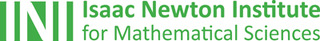## The Isaac Newton Institute: Creating eureka momentsOne of the most exciting places in the mathematical world is the Isaac Newton Institute for Mathematical Sciences (INI), an international research centre and our neighbour here on the University of Cambridge's maths campus.
The INI attracts leading mathematical scientists from all over the world, and is open to all. We are proud to be collaborating with the INI to bring the cutting edge mathematics that is being done there to the general public. The following content is part of this collaboration.

Black Heroes of Mathematics 2021

This inspiring conference featured speakers from Africa, the US, the Caribbean and the UK. Here are some highlights.

Keeping people safe at large events

How can sporting events, concerts, or festivals be kept safe in the face of rising COVID-19 infections?

Maths in a Minute: Category theory

A quick introduction to an abstract concept that's useful in anything from genetics to cosmology.

Opening the black box

The organisers of a new INI programme explain why we need to know more about the maths behind machine learning and deep neural networks.

Maths in a minute: Semi-supervised machine learning

Machine learning started with supervised learning and us providing all the training materials, but we are finding ways for algorithms to learn with far fewer resources.

Maths in a minute: The prime number theorem

A quick look at one of the most important theorems in number theory.

COVID-19 and universities: What do we know?

What can last year's experiences tell us about the coming academic year?

Learning the mathematics of the deep

Explore the maths behind machine learning and deep neural networks with this collection of short introductions and in-depth articles.

Maths in a minute: Gradient descent algorithms

Whether you're lost on a mountainside, or training a neural network, you can rely on the gradient descent algorithm to show you the way!

Maths in a minute: Machine learning and neural networks

Machine learning makes many daily activities possible, but how does it work?

Maths in a minute: Artificial neurons

When trying to build an artificial intelligence, it makes sense to mimic the human brain. Artificial neurons do just that.

Complex square roots

How to take the square root of a complex number — and discover a beautiful new surface in the process.

• Want facts and want them fast? Our Maths in a minute series explores key mathematical concepts in just a few words.